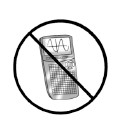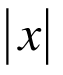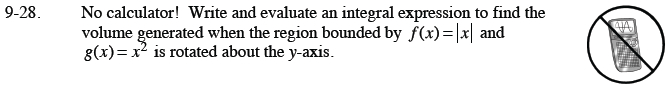### Home > CALC > Chapter 9 > Lesson 9.1.2 > Problem9-28

9-28.

No calculator! Write and evaluate an integral expression to find thevolume generated when the region bounded by f(x) =and g(x) = x2 is rotated about the y-axis. Homework Help ✎Note: The following steps outline the washer method. The answer in the back of the book uses the shell method.

Always start by sketching a graph of the situation.
If you are rotating about the y-axis, will the radii be horizontal or vertical?

Since rotation is about the y-axis, the integral will have dy.
Now, determine the bounds of integration.

Determine which function is the outside function and which is the inside function for the solid.
Then, using the washer method, set up the integral as:

$\int_a^b \pi((r_\text{outide})^2-(r_\text{inside})^2)dy$